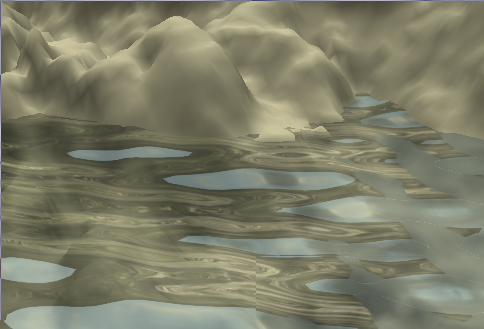# Water simulation in GLSL

Published on 2010-06-02
Edited on 2011-11-26
Tagged: 3d opengl

Over the weekend, I put together a very basic water simulation with GLSL (the shader language in OpenGL). I'm pretty happy with the results so far. It's amazing how much you can get out of such a small amount of code.Step one in generating the water you see above is building a mesh for it. I started with a heightmap, set the "sea level" elevation, and copied everything below that. So the vertices of the mesh actually store depth for the water at every point. I don't use this information right now, but it could probably be used to make smaller or slower waves near the coast for more realism.

The mesh gets drawn with a vertex shader, which essentially applies a sum of sine waves to the surface. The height at each point is a function of XY position and time. The number of waves and the amplitude, wavelength, speed, and direction of each wave are configurable parameters.

```const float pi = 3.14159;
uniform float waterHeight;
uniform float time;
uniform int numWaves;
uniform float amplitude;
uniform float wavelength;
uniform float speed;
uniform vec2 direction;

float wave(int i, float x, float y) {
float frequency = 2*pi/wavelength[i];
float phase = speed[i] * frequency;
float theta = dot(direction[i], vec2(x, y));
return amplitude[i] * sin(theta * frequency + time * phase);
}

float waveHeight(float x, float y) {
float height = 0.0;
for (int i = 0; i < numWaves; ++i)
height += wave(i, x, y);
return height;
}
```

The beautiful thing about modelling wave height as a fixed function like this is that you can generate normal vectors with some simple calculus. Below, I have two functions which take the partial derivatives with respect to X and Y, and another which sums them to produce a normal.

```float dWavedx(int i, float x, float y) {
float frequency = 2*pi/wavelength[i];
float phase = speed[i] * frequency;
float theta = dot(direction[i], vec2(x, y));
float A = amplitude[i] * direction[i].x * frequency;
return A * cos(theta * frequency + time * phase);
}

float dWavedy(int i, float x, float y) {
float frequency = 2*pi/wavelength[i];
float phase = speed[i] * frequency;
float theta = dot(direction[i], vec2(x, y));
float A = amplitude[i] * direction[i].y * frequency;
return A * cos(theta * frequency + time * phase);
}

vec3 waveNormal(float x, float y) {
float dx = 0.0;
float dy = 0.0;
for (int i = 0; i < numWaves; ++i) {
dx += dWavedx(i, x, y);
dy += dWavedy(i, x, y);
}
vec3 n = vec3(-dx, -dy, 1.0);
return normalize(n);
}
```

The normal vector is left in world space so it can be used for cubemap texture coordinates in the fragment shader. I just reused my skybox textures as a cubemap. That's how I got the reflection you see. I set the alpha value for the water to 0.5 so it's fairly transparent.

Here's another screenshot:Exercises left for the reader (and me, next weekend):

• Change transparency based on the angle between the normal vector and the eye vector
• Create a dynamic bump map for high frequency waves, using the same techniques
• Reflection of the environment, not just the skybox
• Refraction so the terrain under the water looks "wavy"